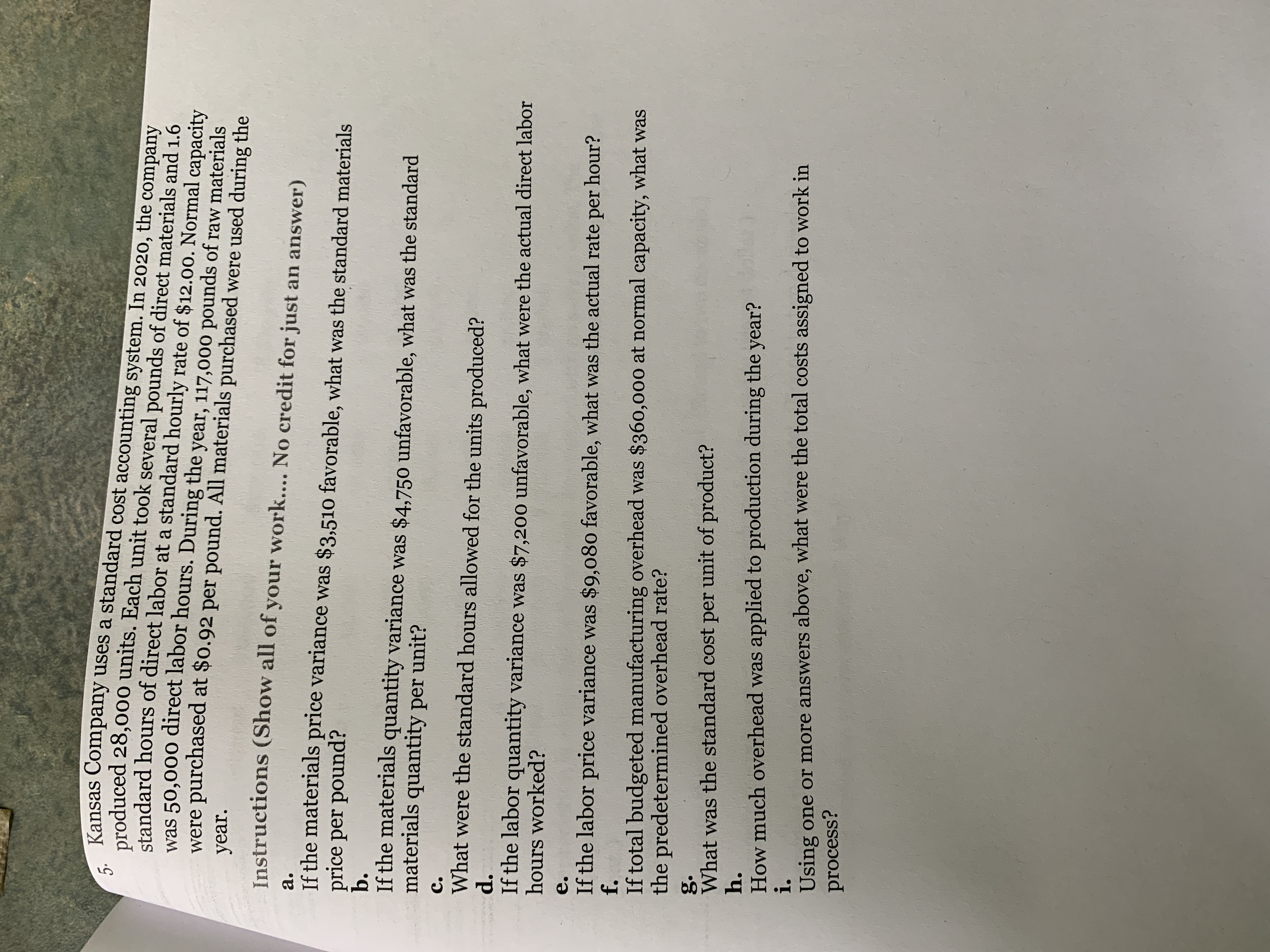# Kansas Company uses a standard cost accounting system. In 2020, the company5.produced 28,000 units. Each unit took several pounds of direct materials and 1.6standard hours of direct labor at a standard hourly rate of \$12.00. Normal capacitywas 50,000 direct labor hours. During the year, 117,000 pounds of raw materialswere purchased at \$0.92 per pound. All materials purchased were used during theyear.Instructions (Show all of your work.... No credit for just an answer)a.If the materials price variance was \$3,510 favorable, what was the standard materialsprice per pound?b.If the materials quantity variance was \$4,750 unfavorable, what was the standardmaterials quantity per unit?C.What were the standard hours allowed for the units produced?d.If the labor quantity variance was \$7,200 unfavorable, what were the actual direct laborhours worked?e.If the labor price variance was \$9,080 favorable, what was the actual rate per hour?f.If total budgeted manufacturing overhead was \$360,000 at normal capacity, what wasthe predetermined overhead rate?g.What was the standard cost per unit of product?h.How much overhead was applied to production during the year?i.Using one or more answers above, what were the total costs assigned to work inprocess?

Question
69 viewshelp_outlineImage TranscriptioncloseKansas Company uses a standard cost accounting system. In 2020, the company 5. produced 28,000 units. Each unit took several pounds of direct materials and 1.6 standard hours of direct labor at a standard hourly rate of \$12.00. Normal capacity was 50,000 direct labor hours. During the year, 117,000 pounds of raw materials were purchased at \$0.92 per pound. All materials purchased were used during the year. Instructions (Show all of your work.... No credit for just an answer) a. If the materials price variance was \$3,510 favorable, what was the standard materials price per pound? b. If the materials quantity variance was \$4,750 unfavorable, what was the standard materials quantity per unit? C. What were the standard hours allowed for the units produced? d. If the labor quantity variance was \$7,200 unfavorable, what were the actual direct labor hours worked? e. If the labor price variance was \$9,080 favorable, what was the actual rate per hour? f. If total budgeted manufacturing overhead was \$360,000 at normal capacity, what was the predetermined overhead rate? g. What was the standard cost per unit of product? h. How much overhead was applied to production during the year? i. Using one or more answers above, what were the total costs assigned to work in process? fullscreen
check_circle

Step 1

Hence, \$0.94 is the standard price per pound. It is obtained by substituting the values in the formula of materials price variance in which material price variance, actual quantity and actual rate is already given.

Step 2

Hence, \$3.99 is the standard material quantity per unit. It is obtained by dividing the standard quantity by units p...

### Want to see the full answer?

See Solution

#### Want to see this answer and more?

Solutions are written by subject experts who are available 24/7. Questions are typically answered within 1 hour.*

See Solution
*Response times may vary by subject and question.
Tagged in